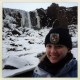# A New Galactic Yardstick

In 1929, Edwin Hubble (yep, the one we named the telescope after) discovered one of the simplest but most surprising relationships in astrophysics. He found that the speed that a galaxy is moving away from us is proportional to its distance ($v = H_{0}*d$) — in other words, the universe is expanding. The constant of proportionality,$H_{0}$, is called the Hubble constant, and it’s a measure of the rate of that expansion.Fig. 1: Author’s impression of an AGN. Part of the torus is cut away so you can see to the central source. Credit: NASA/CXC/M. Weiss

This had a lot of implications for cosmology. In particular, you can roughly calculate the age of the universe from the Hubble constant with$t = 1/H_{0}$. But it’s hard to get an accurate measurement of the Hubble constant. While the velocities of astronomical objects are easy to measure with Doppler shifts, distances are really hard to measure observationally. Check out this astrobite from 2012 for a great discussion of how we calculate distances using standard candles. The basic idea is that if we know how intrinsically bright an object is, we can calculate its distance using its apparent brightness as seen from Earth. A lot of work has been done in finding objects with special characteristics that let us measure the intrinsic brightness without knowing the distance (like Cepheid variable stars or Type Ia supernovae).

The authors of this article propose a new technique for distance measurements using the brightness of active galactic nuclei (AGN). We’ve talked about AGN quite a bit on astrobites, but for the purposes of this article you just need to know that an AGN is made up of a very bright central source surrounding by a torus of dust (see Fig. 1 for an illustration). There’s a region in between the central source and the dust torus that is too hot for dust to exist, and we know that the size of this region is proportional to the brightness of the source. So if we can measure the distance from the source to the dust torus, we can calculate the brightness of the source, which will then tell us the distance of the AGN from Earth.Fig. 2: Plot of the brightness of two AGN vs. time at short wavelengths (light green, in V-band) and longer wavelengths (red, in K-band). The central source emits the short-wavelength light, which the dust re-emits at longer wavelengths a short time later.

The brightness of the central source can vary over time. Short-wavelength light crosses the empty region and is absorbed by the dust at the inner edge of the torus, which re-emits longer-wavelength light with the same pattern of variability but a short time later, like an echo. You can see this light “echo” in Fig. 2. By measuring the time delay of this echo and using the known speed of light, the authors of this paper could calculate the size of the cleared region between the source and the dust torus. This technique is called reverberation mapping and is commonly used to probe the structure of AGN. The authors used reverberation mapping to calculate the brightness of the source, then the distance to the AGN.Fig. 3: Velocity vs. distance for the 17 AGN discussed in this paper (in red) and the Cepheid variable sources from the Hubble Space Telescope Key Project (in light green). The straight black line shows the least-squares fit to the AGN data. The straight black line shows the Hubble equation$v=H_{0} d$ with a Hubble constant of 73 km s-1 Mpc-1.

The authors used this technique on 17 AGN observed by the Multicolor Active Galactic Nuclei Monitoring (MAGNUM) program at the Haleakala Observatory. Then they looked up the velocities of the AGN on the NASA/IPAC Extragalactic Database and plotted velocity vs. distance to calculate the Hubble constant. Fig. 3 shows their velocity vs. distance measurements as well as measurements from the Hubble Space Telescope Key Project, which measured the distances and velocities of Cepheid variable stars in other galaxies. The authors calculate a Hubble constant of 73 ± 3 km s-1 Mpc-1, which agrees well with the Hubble Key measurements of 75 ± 10 km s-1 Mpc-1, but extends to much farther distances.

This technique provides a new method for measuring cosmological distances that does not depend on any other methods, which gives us an independent (and, incidentally, more accurate) measurement of the Hubble constant that reassuringly agrees with the Cepheid variable measurement. The authors mention two other distance measurement techniques using reverberation mapping of AGN proposed by previous authors, but this is the first method that found a value for the Hubble constant that agrees with the value found by other techniques.